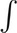# AP Calculus BC Question 62: Answer and Explanation

### Test Information

Question: 62

7. A rock is thrown straight upward with an initial velocity of 50 m/s from a point 100 m above the ground. If the acceleration of the rock at any time t is a = -10 m/s2, what is the maximum height of the rock (in meters)?

• A. 150
• B. 175
• C. 200
• D. 225

Explanation:

D Because the derivative of velocity with respect to time is acceleration, we have

v(t) =- 10 dt = - 10t + C

Now we can plug in the initial condition to solve for the constant.

50 = -10(0) + C

C = 50

Therefore, the velocity function is v(t) = -10t + 50.

Note that the velocity is zero at t = 5.

Next, because the derivative of position with respect to time is velocity, we have

s(t) =(- 10t + 50) dt = - 5t2 + 50t + C

Now, we can plug in the initial condition to solve for the constant.

100 = -5(0)2 + 50(0) + C

C = 100

Therefore, the position function is s(t) = -5t2 + 50t + 100.

The maximum height occurs when the velocity is zero, so we plug t = 5 into the position function to get s(5) = -5(5)2 + 50(5) + 100 = 225.